# Introduction to cnbdistr

#### July 2017

cnbdistr provides functions for working with the conditional distribution of $$X$$ given $$X + Y$$, where $$X$$ and $$Y$$ are independent of each other, drawn from two Negative Binomials that are alllowed to have completely different parameters. I refer to this new distribution as the Conditional Negative Binomial ($$\text{CNB}$$).

Many real-world applications involve overdispersed count data that can be adequately modeled by Nagative Binomials ($$\text{NB}$$). Hence I expect the $$\text{CNB}$$ described above to be useful in such scenarios when we need to model the conditional counts. Having derived its probability mass function (PMF), distribution function (CDF) and moments in closed analytic form, as well as some other aspects of the $$\text{CNB}$$, I decided to implement these in cnbdistr. In particular, quantile function and random generator have been implemented. Here is a list of functions currently available in cnbdistr:

• dcnb and qcnb: PMF and CDF.
• mu_cnb and sigma2_cnb: first and second central moment.
• qcnb and rcnb: quantile function and random generator.

## Probability Model

Assume $$X$$ and $$Y$$ are independent random variables such that

$X \sim \text{NB}(r_1, p_1) \quad \text{ i.e.,} \quad f_X(k)=\binom{k + r_1 - 1}{k}\cdot(1 - p_1)^{r_1}p_1^{k}$ $Y \sim \text{NB}(r_2, p_2) \quad \text{ i.e.,} \quad f_Y(k)=\binom{k + r_2 - 1}{k}\cdot(1 - p_2)^{r_2}p_2^{k}$

,then it can be shown that the conditional distribution of $$X$$ given $$X + Y = D$$ depends on $$\{p_1, p_2\}$$ only through $$p_1/p_2$$:

$X|_{X + Y = D} \sim \text{CNB}\left(D, r_1, r_2, \frac{p_1}{p_2}\right)$

As a special case when $$p_1 = p_2$$, we note that

$$\text{CNB}\left(D, r1, r2, \frac{p_1}{p_2}\right)$$ is identical to $$\text{BB}(D, r1, r2)$$, whose PMF is $$f(k) = \binom{D}{k}\frac{\text{B}(k + r_1, D - k + r_2)}{\text{B}(r_1, r_2)}$$, where $$\text{BB}$$ stands for Beta Binomial distribution, and $$\text{B}$$ stands for Beta function.

Also we note the symmetry:

$Y|_{X + Y = D} \sim \text{CNB}\left(D, r_2, r_1, \frac{p_2}{p_1}\right)$

## PMF

Utilizing Gauss hypergeometric function $${}_2 \text{F}_1$$ (Abramowitz, Stegun, and others 1972), the PMF of $$\text{CNB}\left(D, r1, r2, \frac{p_1}{p_2}\right)$$ can be written in closed form as

$f_{X|_{X+Y=D}}(k) = \frac{\binom{D}{k}\cdot\text{B}(k + r_1, D - k + r_2)\cdot(p_1/p_2)^k}{\text{B}(r_1, r_2 + D) \cdot{}_2 \text{F}_1\left(\begin{matrix}-D,\quad r_1\\-D-r_2+1 &\end{matrix};\frac{p_1}{p_2}\right)}$

With this PMF, the domain of $$r_1$$ and $$r_2$$ can be extended from positive integers to any positive real numbers. In cnbdistr the PMF can be computed by dcnb.

library(cnbdistr)
D <- 11
r1 <- 4
r2 <- 0.8
theta <- 0.63 # this is p1/p2
x <- dcnb(0:D, D, r1, r2, theta)
plot(0:D, x, type='h', lwd=8, col = "palegreen3")
y <- dcnb(0:D, D, r2, r1, 1/theta)
plot(0:D, y, type='h', lwd=8, col = "royalblue3")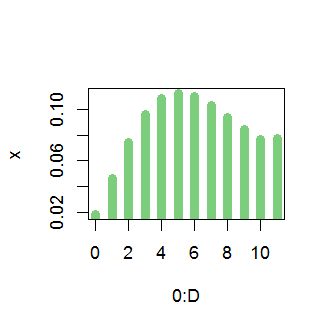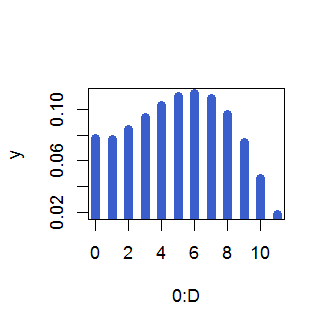## CDF

Utilizing both $${}_2 \text{F}_1$$ and $${}_3 \text{F}_2$$, the CDF of $$\text{CNB}\left(D, r1, r2, \frac{p_1}{p_2}\right)$$ can be written in closed form as

$\sum\limits_{u=0}^{k} f_{X|_{X+Y=D}}(u) = 1 - \frac{{}_3 \text{F}_2\left(\begin{matrix}1, r_1+k+1, -D+k+1\\k+2, -D+k+2-r_2 &\end{matrix};\frac{p_1}{p_2}\right)\cdot\text{B}(k + r_1 + 1, D - k + r_2 - 1)\cdot\left(\frac{p_1}{p_2}\right)^{k+1}}{\text{B}(k + 2, D - k)\cdot(D + 1)\cdot\text{B}(r_1, r_2 + D) \cdot{}_2 \text{F}_1\left(\begin{matrix}-D,\quad r_1\\-D-r_2+1 &\end{matrix};\frac{p_1}{p_2}\right)}$

In cnbdistr the CDF can be computed by pcnb.

library(cnbdistr)
D <- 11
r1 <- 4
r2 <- 0.8
theta <- 0.63 # this is p1/p2
x <- pcnb(0:D, D, r1, r2, theta)
plot(0:D, x, type='s', lwd=4, col = "palegreen3")
y <- pcnb(0:D, D, r2, r1, 1/theta)
plot(0:D, y, type='s', lwd=4, col = "royalblue3")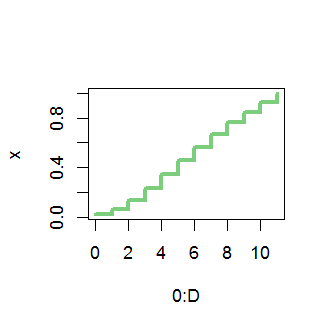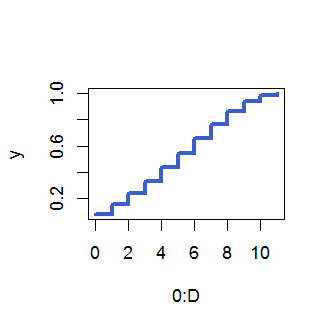## Quantile Function

The quantile function of $$\text{CNB}\left(D, r1, r2, \frac{p_1}{p_2}\right)$$ is defined as

$Q(x) = \inf\limits_{k\in 0:D}\left\{\sum\limits_{u=0}^{k} f_{X|_{X+Y=D}}(u) \geq x\right\}$

and it is implemented by applying bisection method to the distribution function. In cnbdistr the quantile function can be computed by qcnb.

library(cnbdistr)
D <- 11
r1 <- 4
r2 <- 0.8
theta <- 0.63 # this is p1/p2
x <- qcnb(seq(0, 1, 0.01), D, r1, r2, theta)
plot(seq(0, 1, 0.01), x, type='s', lwd=4, col = "palegreen3")
y <- qcnb(seq(0, 1, 0.01), D, r2, r1, 1/theta)
plot(seq(0, 1, 0.01), y, type='s', lwd=4, col = "royalblue3")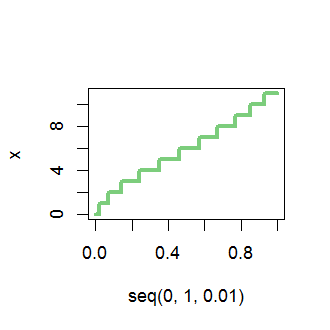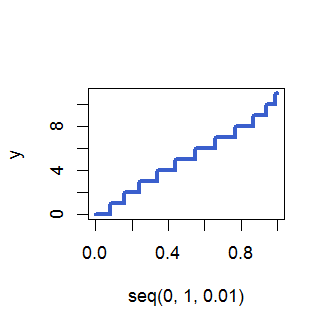## Random Generator

For drawing samples at random from $$\text{CNB}\left(D, r1, r2, \frac{p_1}{p_2}\right)$$, a random generator has been implemented by applying inverse transform sampling to the quantile function. In cnbdistr the random generation is carried out by rcnb.

library(cnbdistr)
D <- 11
r1 <- 4
r2 <- 0.8
theta <- 0.63 # this is p1/p2
x <- rcnb(1e4, D, r1, r2, theta)
hist(x, seq(-0.5, 0.5 + D, by = 1), col = 'gray72')
table(x) / 1e4
## x
##      0      1      2      3      4      5      6      7      8      9
## 0.0190 0.0461 0.0728 0.1001 0.1071 0.1078 0.1113 0.1024 0.0947 0.0817
##     10     11
## 0.0771 0.0799
format(dcnb(0:D, D, r1, r2, theta), digits=2)
##   "0.018" "0.046" "0.074" "0.096" "0.108" "0.112" "0.110" "0.103"
##   "0.094" "0.084" "0.077" "0.077"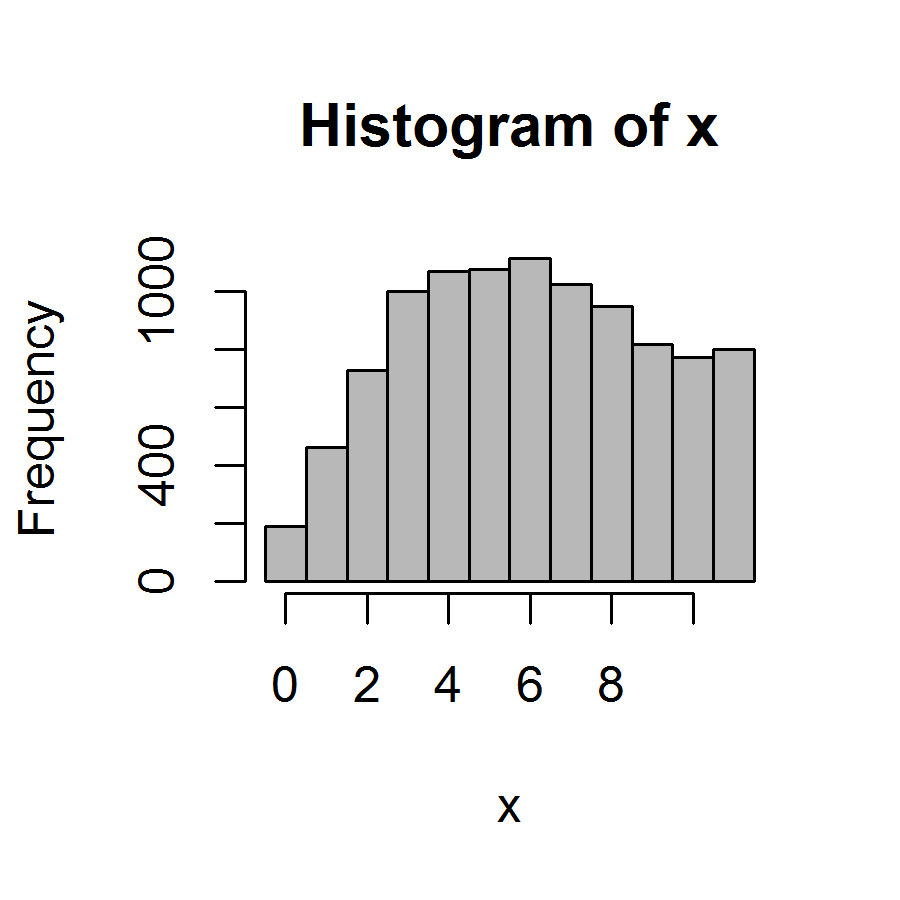## Moments

Mean of $$\text{CNB}\left(D, r1, r2, \frac{p_1}{p_2}\right)$$ is given by

$E(X|_{X+Y=D}) = D\cdot\frac{p_1}{p_2}\cdot\frac{r_1}{r_2 + D - 1}\cdot\frac{{}_2 \text{F}_1\left(\begin{matrix}-D+1,\quad r_1+1\\-D-r_2+2 &\end{matrix};\frac{p_1}{p_2}\right)}{{}_2 \text{F}_1\left(\begin{matrix}-D,\quad r_1\\-D-r_2+1 &\end{matrix};\frac{p_1}{p_2}\right)}$

library(cnbdistr)
D <- 11
r1 <- 4
r2 <- 0.8
theta <- 0.63 # this is p1/p2
mu_cnb(D, r1, r2, theta)
##  5.984561
sum(c(0:D) * dcnb(0:D, D, r1, r2, theta))
##  5.984561

Variance of $$\text{CNB}\left(D, r1, r2, \frac{p_1}{p_2}\right)$$ is given by

$V(X|_{X+Y=D}) = D(D-1)\cdot\left(\frac{p_1}{p_2}\right)^2\cdot\frac{r_1(r_1+1)}{(r_2 + D - 1)(r_2+D-2)}\cdot\frac{{}_2 \text{F}_1\left(\begin{matrix}-D+2,\quad r_1+2\\-D-r_2+3 &\end{matrix};\frac{p_1}{p_2}\right)}{{}_2 \text{F}_1\left(\begin{matrix}-D,\quad r_1\\-D-r_2+1 &\end{matrix};\frac{p_1}{p_2}\right)} \\ + E(X|_{X+Y=D})\left(1-E(X|_{X+Y=D})\right)$

library(cnbdistr)
D <- 11
r1 <- 4
r2 <- 0.8
theta <- 0.63 # this is p1/p2
sigma2_cnb(D, r1, r2, theta)
##  8.795583
sum((c(0:D) - mu_cnb(D, r1, r2, theta))^2 * dcnb(0:D, D, r1, r2, theta))
##  8.795583